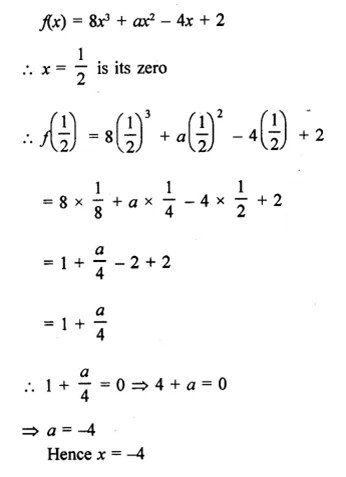• +91 9971497814
• info@interviewmaterial.com

# RD Chapter 6- Factorisation of Polynomials Ex-VSAQS Interview Questions Answers

### Related Subjects

Question 1 : Define zero or root of a polynomial.

Answer 1 : A real number a is a zero or root of a polynomial f(x) if f(α) = 0

Question 2 : If x = 12 is a zero of the polynomial f(x) =  8x3 +ax2 – 4x + 2, find the value of a.Question 3 : Write the remainder whenthe polynomial f(x) = x3+x2-3x + 2is divided by x + 1.

f(x) = x3+x2-3x + 2
Let x + 1 = 0, then x = -1
Remainder =(-1)
Now,f(-1) = (-1)3 + (-1)2 – 3(-1) + 2
= -1 + 1+ 3 + 2 = 5
Remainder = 5

Question 4 : Find the remainder when x3 +4x2 + 4x – 3 is divided by x.

f(x) = x3 + 4x2 + 4x – 3
Dividing f(x) by x, we get
Let x = 0, then
f(x) = 0 + 0 + 0 – 3 = -3
Remainder= -3

Question 5 : If x + 1 is a factor of x3 +a, then write the value of a.

Let f(x) = x3 + a
x + 1is a factor of fx)
Let x + 1 = 0
x = -1
f(-1)= x3 + a
= (-1)3 + a = -1 + a
x + 1 is a factor
Remainder = 0
-1 + a = 0  a = 1
Hence a = 1

Question 6 : If f(x) = x4-2x3 +3x2 – ax – b when divided by x – 1, the remainder is 6, thenfind the value of a + b.

f(x) = x4 – 2x3 + 3x2 –ax – b
Dividing f(x) by x – 1, the remainder = 6
Now let x – 1 = 0, then x = 1
f(1) = (1)4 – 2(1)3 + 3(1)2 -ax1-b
= 1 -2 + 3-a-b = 2-a-b
Remainder = 6
2 – a– b = 6  a + b= 2 – 6 = -4
Hence a + b = -4

Todays Deals### RD Chapter 6- Factorisation of Polynomials Ex-VSAQS Contributorskrishan

Name:
Email:

# Latest News# 9000 interview questions in different categories